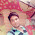## Wednesday, 27 June 2012

### CBSE Class 11 - Physics - Units and Measurements - MCQs

Multiple Choice Questions

Q1: Light year is a unit of
(a) time
(b) distance
(c) sunlight intensity
(d) mass

Q2: The dimensional formula for Planck's constant is
(a) [MLT]
(b) [ML2T-1]
(c)  [M2L2T-1]
(d)  [ML1T-1]

Q3: The surface tension of a liquid is 70 dyne/cm. In MKS system its value is?
(a) 70 N/m
(b) 7 ✕ 10-2 N/m
(c) 7 ✕ 102 N/m
(d) 7 ✕ 103 N/m

Q4: The dimensions of Kinetic energy is same as that of
(a) Force
(b) Pressure
(c) Work
(d) Momentum

Q5: At 4° C, the density of water is equal to
(a) 10-3 kg m-3
(b) 10-2 kg m-3
(c) 10 kg m-3
(d) 103 kg m-3

Q6: One watt hour contains how many joules?
(a) 3.6 ✕ 108 J
(b) 3.6 ✕ 102 J
(c) 3.6 ✕ 103 J
(d) 10-3 J

Q7: Which of the following pairs has the same dimensions?
(a) Specific Heat and Latent Heat
(b) Impulse and Momentum
(c) Surface Tension and Force
(d) Moment of Inertia and Torque

Q8: The equation of state of some gases can be expressed as Vander wal equation i.e.
(P + a/v2)(V - b) = RT
Where P is the pressure, V is the volume, T is the absolute temperature and a, b, R are
constants. The dimensions of ‘a’ are:
(a)  [M1L1T-1]
(b)  [M1L-5T1]
(c)  [M2L5T-1]
(d)  [M1L5T-2]

Q9: Electron volt is a unit of
(a) Charge
(b) Potential difference
(c) Energy
(d) Magnetic Force

Q10: There are 20 divisions in 4 cm of the main scale. The vernier scale has 10 divisions. The least count of the instrument is
(a) 0.05 cm
(b) 0.5 cm
(c) 5.0 cm
(d) 0.005 cm

1. (b) distance
2. (b) [ML2T-1]   (Hint use E = hν)
3. (b) 7 ✕ 10-2 N/m
4. (c) Work
5. (d) 103 kg m-3
6.  (c) 3.6 ✕ 103 J
7.  (b) Impulse and Momentum
8.  (d)  [M1L5T-2]
9.  (c) Energy
10. (d) 0.005 cm1.2.I have put ossom video lectures of 11th and 12th class Physics at www.visionnotes.com
you can all share and see and get the free help available

3.Nice questions

4.There should be a solutions for numericals

1.This comment has been removed by the author.